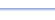Program: SUMATOB

SUMATOB

• Finds the sum of the integers from A to B.
• Finds the sum of N consecutive integers.

Useful for:

• SAT
• ACT
• Algebra 1
• Algebra 2
• Precalculus
• Calculus

See full list of programs

Example 1

Q: Find the sum of the integers from 5 to 42, inclusive.

Solution:

Run SUMATOB.  How?

Choose 1 for
'From A to B'

Enter
A=5, B=42

The program reports:

Interpretation:
The sum is 893.

See Example 2